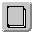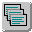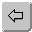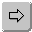#### Date and Time Functions

The following table lists the date and time functions that ODBC supports.

The date and time functions listed can take the following arguments:

• date_exp can be a column name, a date or timestamp literal, or the result of another scalar function, where the underlying data type can be represented as SQL_CHAR, SQL_VARCHAR, SQL_DATE, or SQL_TIMESTAMP.

• time_exp can be a column name, a timestamp or timestamp literal, or the result of another scalar function, where the underlying data type can be represented as SQL_CHAR, SQL_VARCHAR, SQL_TIME, or SQL_TIMESTAMP.

• timestamp_exp can be a column name, a time, date, or timestamp literal; or the result of another scalar function, where the underlying data type can be represented as SQL_CHAR, SQL_VARCHAR, SQL_TIME, SQL_DATE, or SQL_TIMESTAMP.

Function Returns
CURRENT_DATE() Current date.
CURRENT_TIME[(time-precision)] Current local time. The time-precision argument determines the seconds precision of the returned value.
CURRENT_TIMESTAMP[(timestamp-precision)] Current local date and local time as a timestamp value. The timestamp-precision argument determines the seconds precision of the returned timestamp.
CURDATE() Current date as a date value.
CURTIME() Current local time as a time value.
DAYNAME(date_exp) Character string containing a data-source-specific name of the day for the day portion of date_exp.
DAYOFMONTH(date_exp) Day of the month in date_exp as an integer value.
DAYOFWEEK(date_exp) Day of the week in date_exp as an integer value.
DAYOFYEAR(date_exp) Day of the year in date_exp as an integer value.
HOUR(time_exp) Hour in time_exp as an integer value.
MINUTE(time_exp) Minute in time_exp as an integer value.
MONTH(date_exp) Month in date_exp as an integer value.
MONTHNAME(date_exp) Character string containing the data source-specific name of the month.
NOW() Current date and time as a timestamp value.
QUARTER(date_exp) Quarter in date_exp as an integer value.
SECOND(time_exp) Second in time_exp as an integer value.
TIMESTAMPADD(interval, integer_exp, time_exp) Timestamp calculated by adding integer_exp intervals of type interval to time_exp. The interval can be SQL_TSI_FRAC_SECOND, SQL_TSI_SECOND, SQL_TSI_MINUTE, SQL_TSI_HOUR,SQL_TSI_DAY, SQL_TSI_WEEK, SQL_TSI_MONTH, SQL_TSI_QUARTER, or SQL_TSI_YEAR

Fractional seconds are expressed in billionths of a second.

TIMESTAMPDIFF(interval, time_exp1, time_exp2) Integer number of intervals of type interval by which time_exp2 is greater than time_exp1. interval has the same values as TIMESTAMPADD. Fractional seconds are expressed in billionths of a second.
WEEK(date_exp) Week of the year in date_exp as an integer value.
YEAR(date_exp) Year in date_exp. The range is data-source dependent.